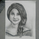117 views
117
I find USDINR is preparing for small up movement. So did one full Elliot wave analysis.

When the USDINR Elliot wave analysis was complete, result was not satisfying.

But The correct cycles are ((I)), ((II)), and (( III )) marked on chart, And I tried to fit all five which went wrong and waste of time.

This analysis is for reference purpose and learning only.

Finally - This analysis is not validating as per basic Elliot wave rules

The Reason : I decided the Grand cycles ((I)), ((II)) .... ((V)) first and then tried to fix the subwaves.

Correction: While putting the first Grand cycle ((I)), the subwaves should fit and then only should go next to mark ((II)) and so on.can you attempt once more a wave count on a weekly chart ?